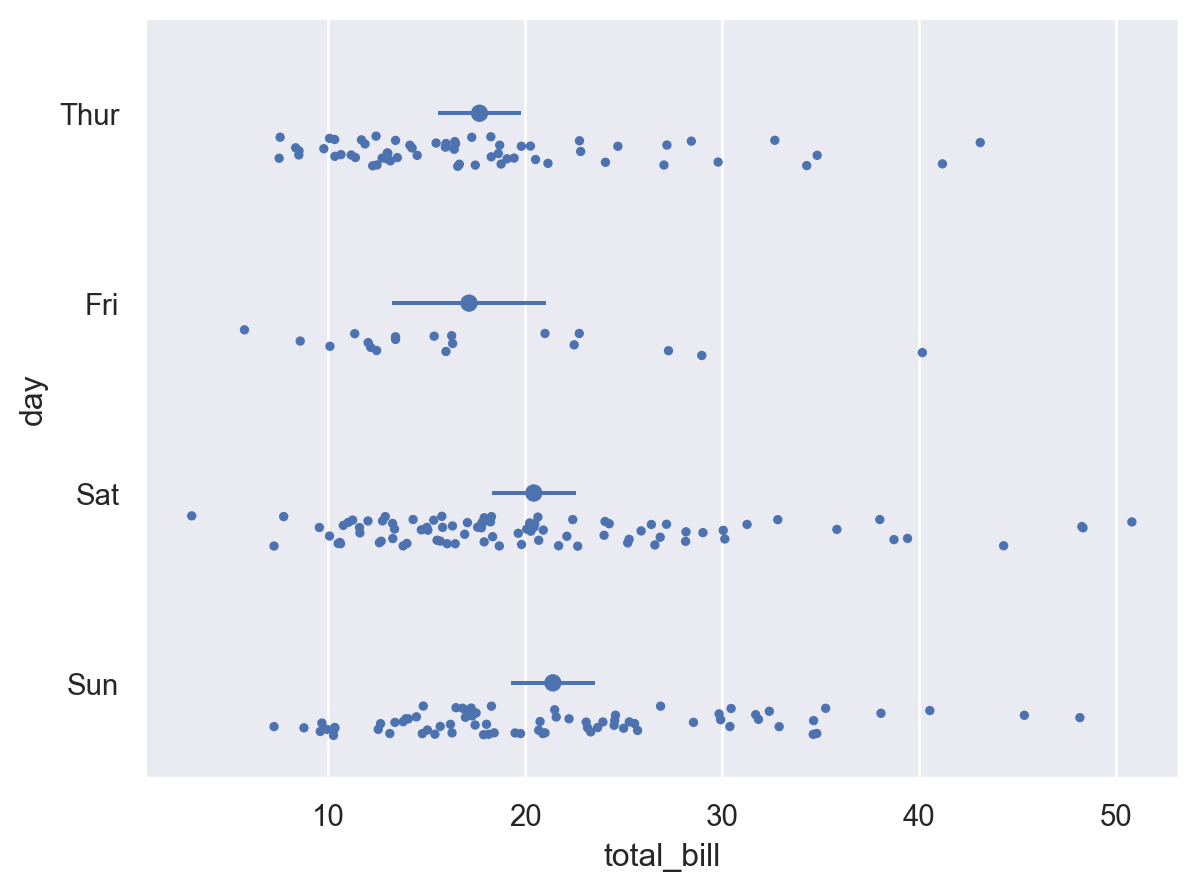# seaborn.objects.Dot#

class seaborn.objects.Dot(artist_kws=<factory>, marker=<'o'>, pointsize=<6>, stroke=<0.75>, color=<'C0'>, alpha=<1>, fill=<True>, edgecolor=<depend:color>, edgealpha=<depend:alpha>, edgewidth=<0.5>, edgestyle=<'-'>)#

A mark suitable for dot plots or less-dense scatterplots.

This mark defines the following properties:

`Dots`

A dot mark defined by strokes to better handle overplotting.

Examples

This mark draws relatively large, filled dots by default:

```p1 = so.Plot(tips, "total_bill", "tip")
```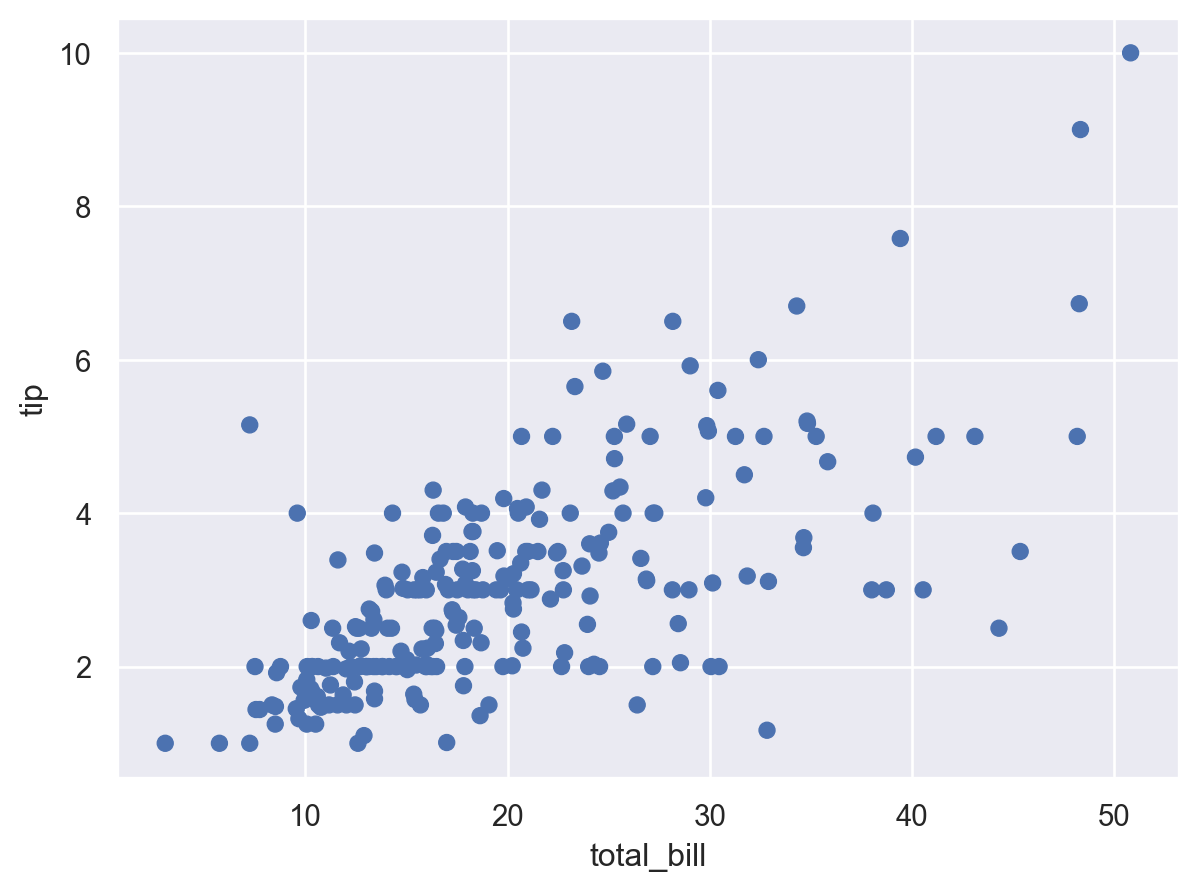While `Dots` is a better choice for dense scatter plots, adding a thin edge can help to resolve individual points:

```p1.add(so.Dot(edgecolor="w"))
```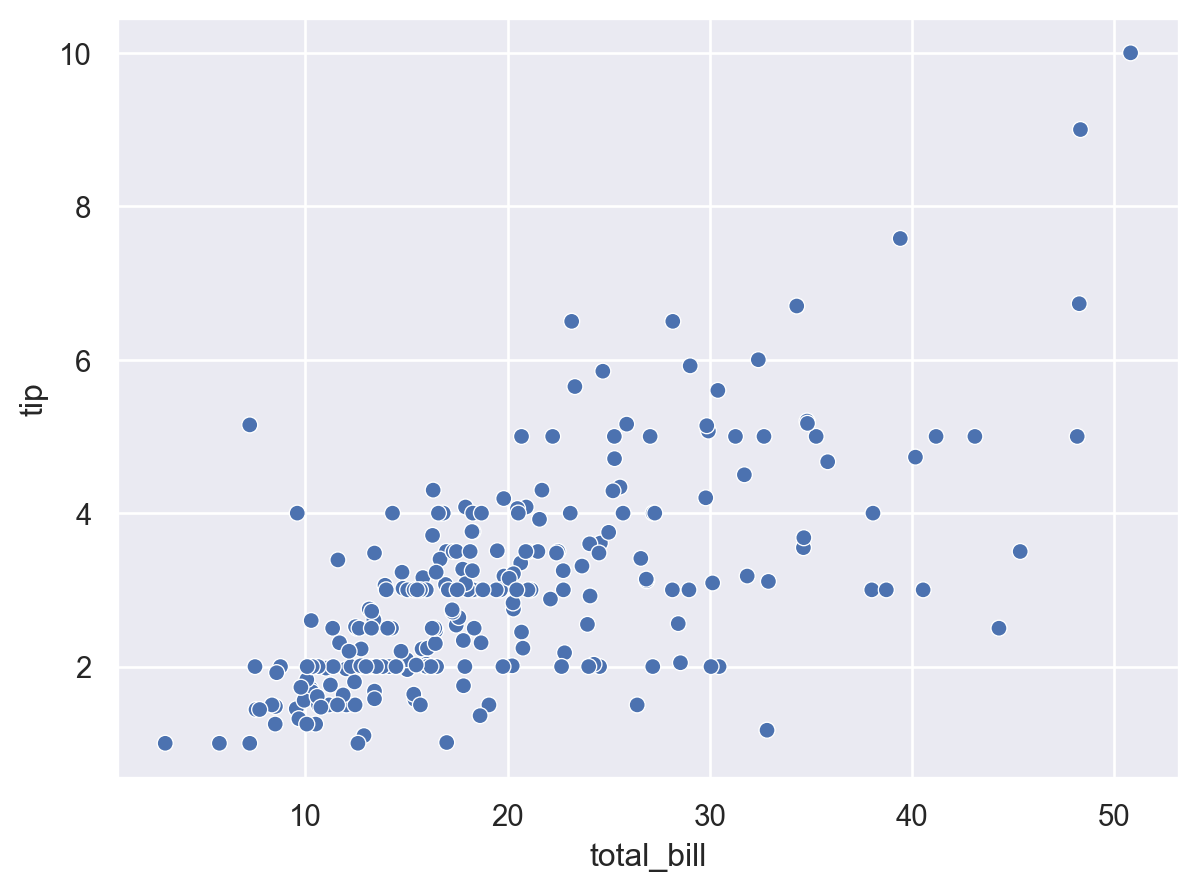Dodging and jittering can also help to reduce overplotting, when appropriate:

```(
so.Plot(tips, "total_bill", "day", color="sex")
)
```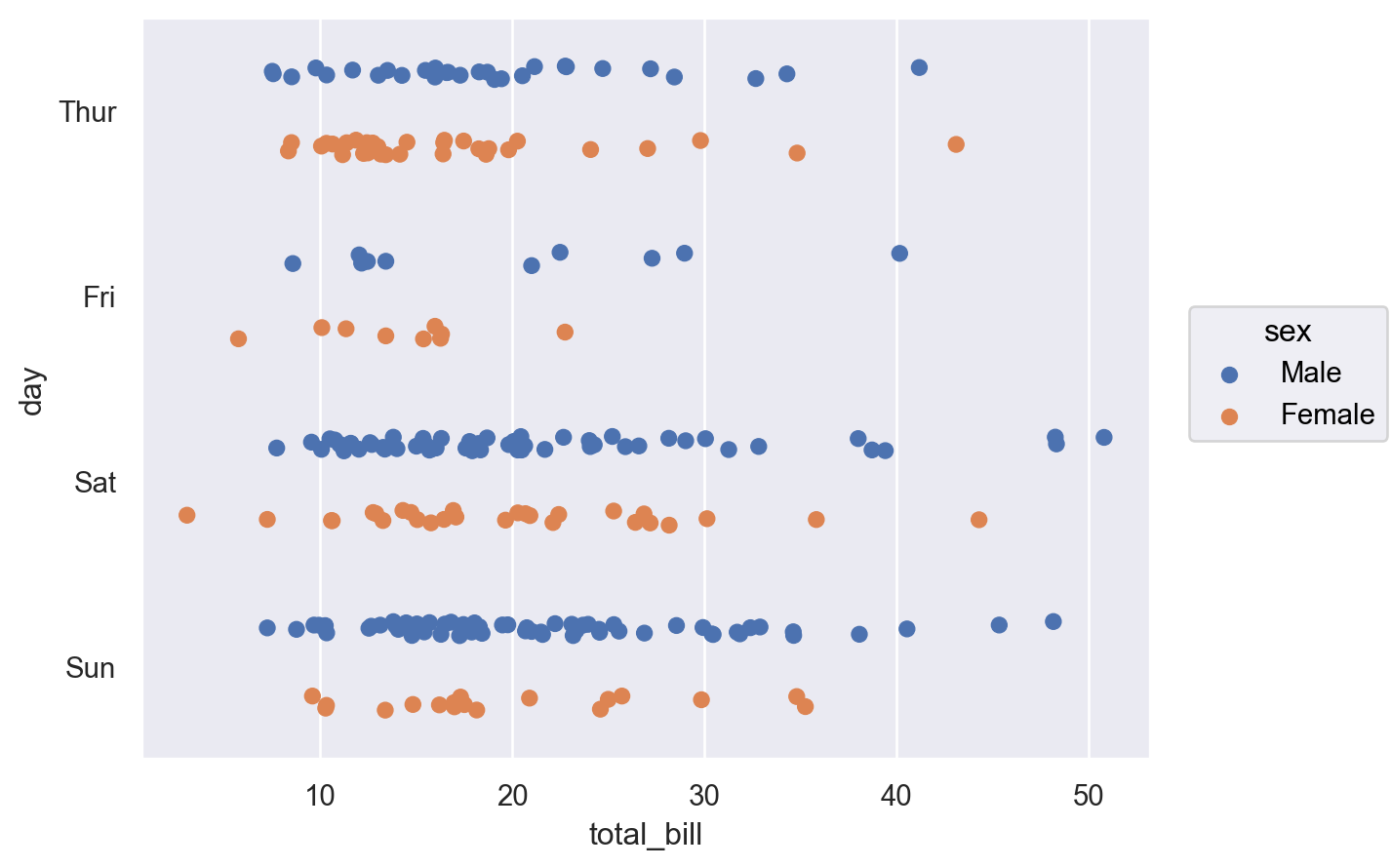The larger dot size makes this mark well suited to representing values along a nominal scale:

```p2 = so.Plot(glue, "Score", "Model").facet("Task", wrap=4).limit(x=(-5, 105))
```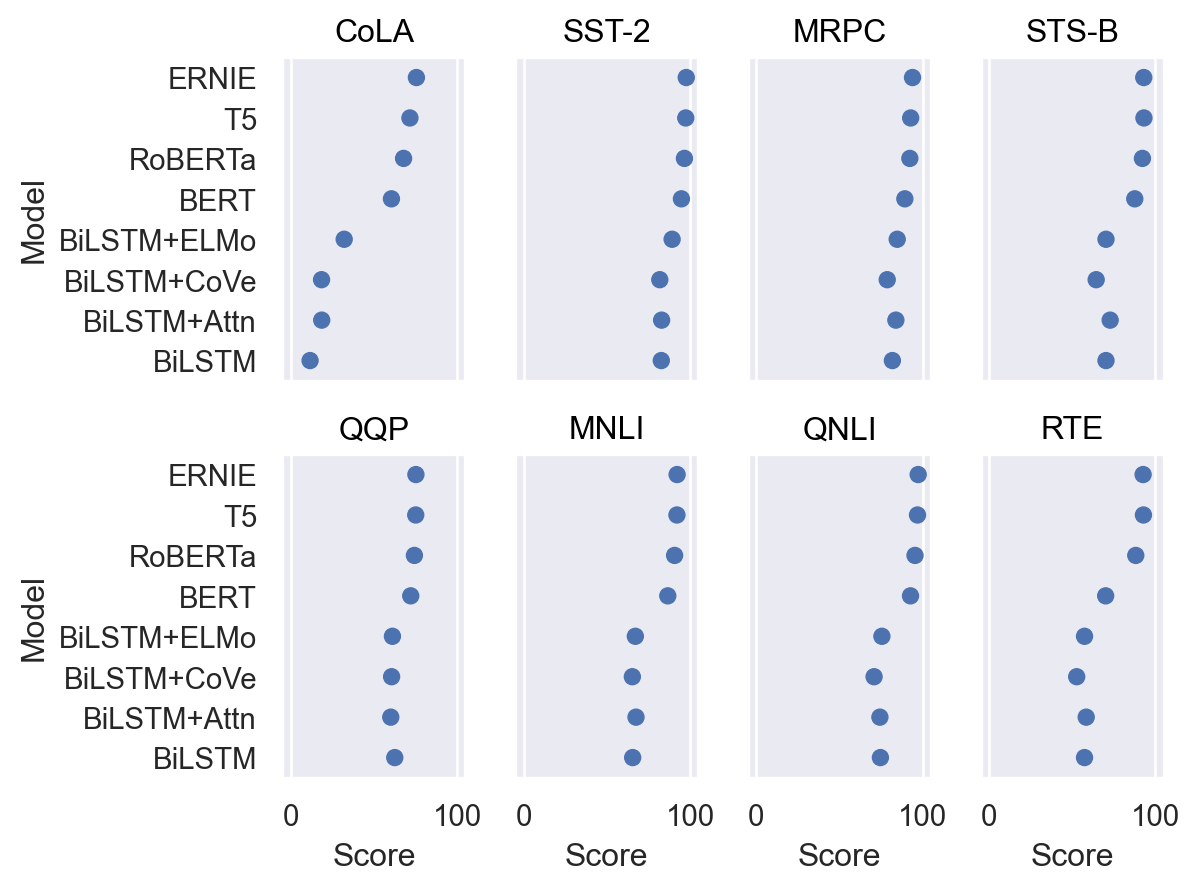A number of properties can be set or mapped:

```(
p2
.scale(marker=["o", "s"], color="flare")
)
```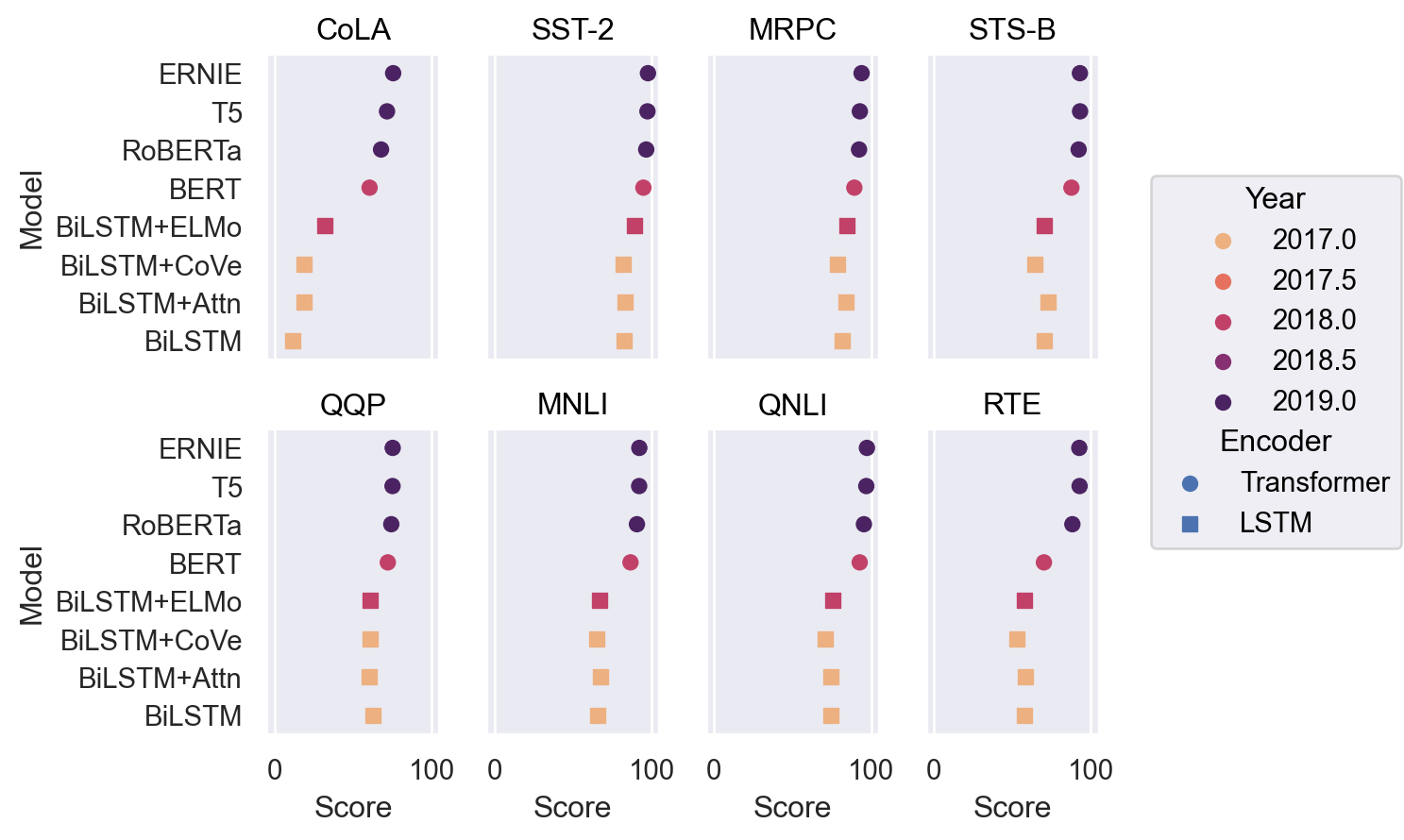Note that the edge properties are parameterized differently for filled and unfilled markers; use `stroke` and `color` rather than `edgewidth` and `edgecolor` if the marker is unfilled:

```p2.add(so.Dot(stroke=1.5), fill="Encoder", color="Encoder")
```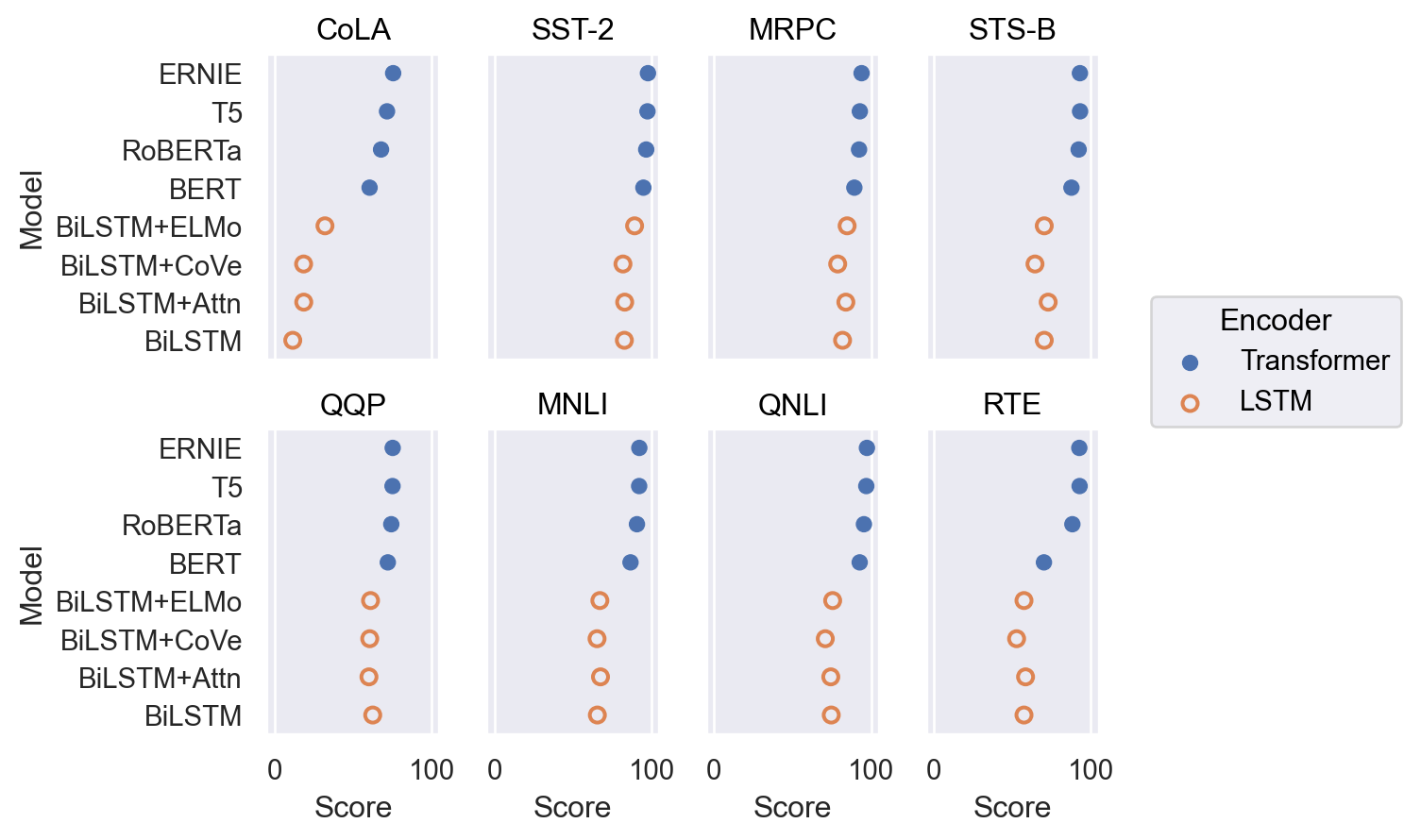Combine with `Range` to show error bars:

```(
so.Plot(tips, x="total_bill", y="day")